Next: Polarization Up: Beamstrahlung Previous: Basic formulas

### Algorithm of event generation

The random number generation using the acception-rejection method is applicable when the distribution function is everywhere finite and is most efficient when the function is flat.

Since the function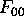is infinite at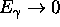, the following variable y is introduced in CAIN instead of the photon energy fraction x in order to make the distribution function finite and relatively flat.The number of photons during a time interval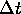in the photon energy range (y,y+dy) is then given by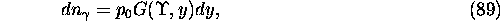where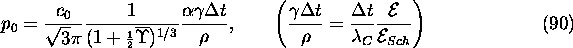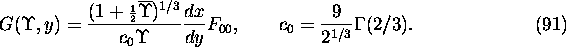The function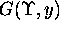is less than or equal to unity for any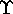and y. It is plotted in Fig.7.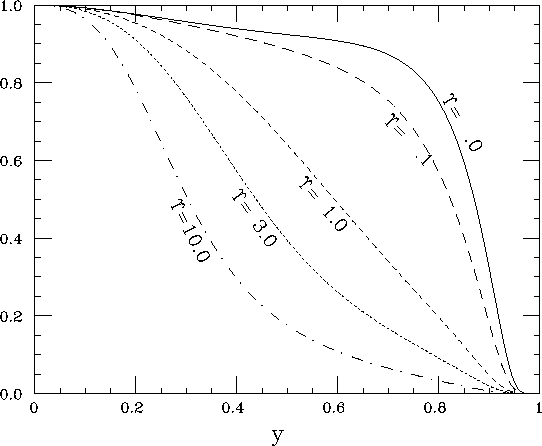Figure 7: Functionfor various values of. Unpolarized case only.

The photon generation in CAIN proceeds in the following way.

(1)
Calculate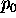for given field, electron energy, and time interval.
(2)
Generate one random number p which is uniform in (0,1).
(3)
If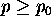, reject emitting a photon. Otherwise,
(4)
Generate one more random number y uniform in (0,1).
(5)
Calculate. (A polynomial approximation is used for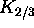and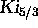. The relative error is less than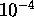.)
(6)
If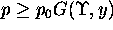, reject emitting a photon. Otherwise,
(7)
Emit a photon whose energy is given by eq.(88).
The cases when accepted in (3) but rejected in (6) cause a waste of time because the calculation ofis the most time consuming. The probability to be accepted in (6) is plotted in Fig.8 is given by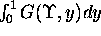and is plotted as a function of. One finds the probability is very high for anyowing to the choice of the variable y.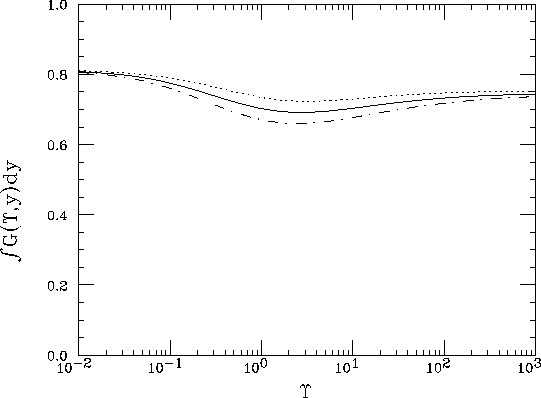Figure 8: The acception probability in the step (6) as a function of. The solid line is the unpolarized case The dot-dash and dotted lines are polarized cases with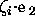= 1 and -1, respectively.Next: Polarization Up: Beamstrahlung Previous: Basic formulas

Toshiaki Tauchi
Thu Dec 3 17:27:26 JST 1998